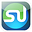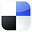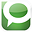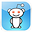# Using the Normal Distribution in Excelto Find Sales Limits

This article will show you exactly how to use the Normal Distribution in Excel to calculate the probability that your daily sales will fall below a certain limit. The advantages of statistics in Excel to solve business statistics problems is that most problems can be solved in just one or two steps and there is no more need to look anything up on Normal Distribution tables.

As with any statistical test that uses or depends on the Normal Distribution, as assumption is made that your sales data is Normally Distributed. If you have at least 30 days of sample data, this is probably a reasonable assumption. Even so, you should always test for Normality prior to performing any parametric test or analysis.

Parametric means using or depending on a statistical distribution such as the Normal distribution. This blog contains a number of articles about Normality tests and nonparametric tests that are used in the event that your data is not Normally distributed.

In this problem, you are provided with the mean and standard deviation of your daily sales data and are then required to find the probability your sales from any random day will fall below a given limit. Here is the problem:

Problem: A store has normally distributed daily sales. We know this because we have tested the distribution of sales results for Normality (to see how this is done, check out recent articles in this blog). The average daily sales = \$2,000 and the daily sales standard deviation = \$500, What is the probability that the sales during one day will fall below \$1,000?

Since we are solving for a probability (area under the Normal curve to the left of X = 1000) for a Normal curve that is not standardized, we will use NORMDIST instead of NORMSDIST, which is used for a standardized Normal curve. See the Excel graph below to view a graphical interpretation of this business statistics problem.

The standard Normal Distribution has a mean equal to 0 and a standard deviation equal to 1. That will not be the case in this business statistics problem.

The Excel formula is as follows:

NORMDIST (x, Sample Mean, Sample Standard Deviation, Cumulative?) =

Cumulative asks if we are calculating the Cumulative Distribution Function [Yes] or the Probability Density Function [No]

= Probability of a sample drawn being less than x (percentage of area under the Normal Curve to the left of x)(Standardized Normal curve has mean = 0 and standard deviation = 1)

NORMDIST (1000,2000,500,TRUE) = 0.0228

Only 2.28% of the total area under this Normal curve falls to the left of x = 1,000 if the mean = 1,000 and the standard deviation = 500 therefore the probability is only 2.28% that daily sales will fall below 1,000.

Click On Image To See Larger Version

Basic Description of Normal Distribution

The shape of the Normal distribution resembles a bell so it is sometimes called the "bell curve." The Normal curve is symmetric about its mean in the middle, and its tails on either side extend to infinity. The Normal distribution is a continuous function, not a discrete function, This means that any value can be graphed somewhere on a Normal curve. Discrete functions only map specific values, such as whole numbers.

Mapping the Normal Distribution

Any Normal distribution can be completely mapped if only the following two parameters are known:

1) Mean - µ - the Greek symbol "mu"

2) Standard deviation - σ - the Greek symbol "sigma"

If the mean and standard deviation of a Normal distribution are known, then every point of the Normal curve can be mapped.

*************************************************************

A Standardized Normal Curve is a Normal curve that has mean = 0 and standard deviation = 1

*************************************************************

The "68 - 95 - 99.7%" Rule

for the Normal distribution states that:

68% of all observations lie within 1 standard deviation of the mean, within the range of µ +/- σ

95% of all observations lie within 2 standard deviations of the mean, within the range of µ +/- 2σ

99.7% of all observations lie within 3 standard deviations of the mean, within the range of µ +/- 3σ

Click Image To See Larger Version

Click Image To See Larger Version

*****************************************

Using the Normal Distribution to Find Sales Limits

If You Like This, Then Share It...Excel Master Series Blog Directory

Statistical Topics and Articles In Each Topic

• Histograms in Excel
• Bar Chart in Excel
• Combinations & Permutations in Excel
• Normal Distribution in Excel
• t-Distribution in Excel
• Binomial Distribution in Excel
• z-Tests in Excel
• t-Tests in Excel
• Hypothesis Tests of Proportion in Excel
• Chi-Square Independence Tests in Excel
• Chi-Square Goodness-Of-Fit Tests in Excel
• F Tests in Excel
• Correlation in Excel
• Pearson Correlation in Excel
• Spearman Correlation in Excel
• Confidence Intervals in Excel
• Simple Linear Regression in Excel
• Multiple Linear Regression in Excel
• Logistic Regression in Excel
• Single-Factor ANOVA in Excel
• Two-Factor ANOVA With Replication in Excel
• Two-Factor ANOVA Without Replication in Excel
• Creating Interactive Graphs of Statistical Distributions in Excel
• Solving Problems With Other Distributions in Excel
• Optimization With Excel Solver
• Chi-Square Population Variance Test in Excel
• Analyzing Data With Pivot Tables
• SEO Functions in Excel
• Time Series Analysis in Excel

#### 1 comment:

1.This helped a lot! I'm still trying to figure out how to use the NORM.DIST function to find x when the it is the highest 10%.